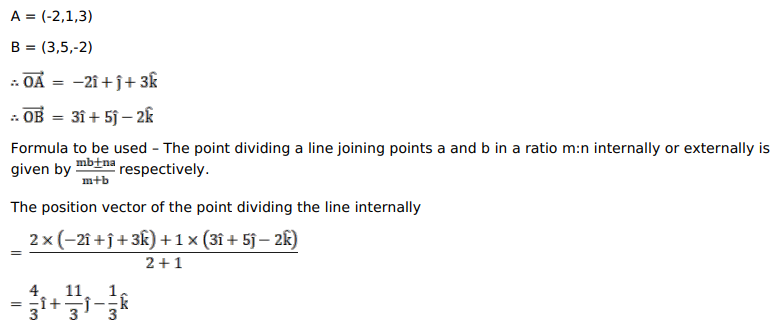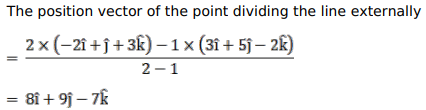# Find the position vector of a point

Question:

Find the position vector of a point $\mathrm{R}$ which divides the line joining $A(-2,1,3)$ and $B(3,5,-2)$ in the ratio $2: 1$ (i) internally (ii) externally.

Solution: